Function Repository Resource:

# SemiEulerianGraphQ

Test if a connected undirected graph is semi-Eulerian

Contributed by: Peter Cullen Burbery
 ResourceFunction["SemiEulerianGraphQ"][g] yields True if the connected undirected graph g is semi-Eulerian and False otherwise.

## Details

A graph is semi-Eulerian if it is possible to traverse the graph crossing each edge exactly once without backtracking or retracing your steps if you can start and stop at different vertices.
ResourceFunction["SemiEulerianGraphQ"] also works for multigraphs.

## Examples

### Basic Examples (3)

Construct a graph with only two odd vertices:

 In:=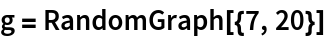Out=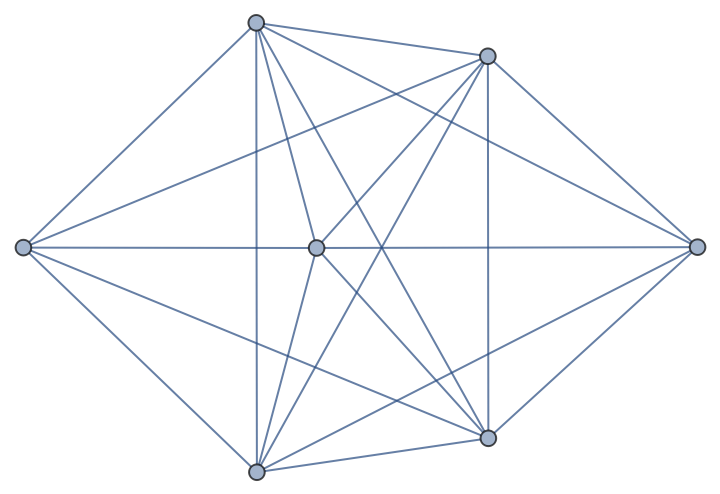In:=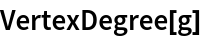Out=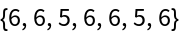Test if the graph is semi-Eulerian:

 In:=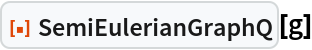Out=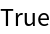Test if the graph is also Eulerian:

 In:=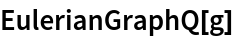Out=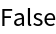A graph with no odd vertices:

 In:=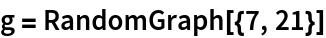Out=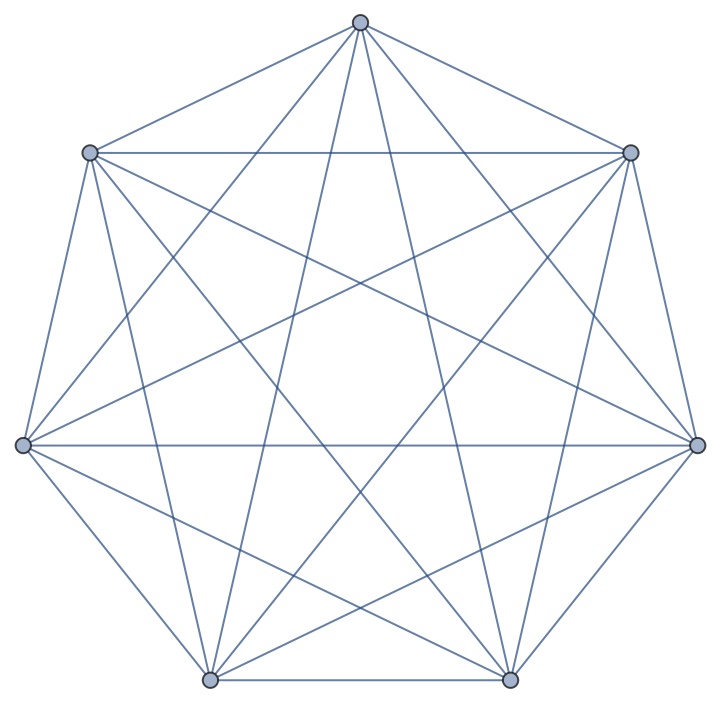In:=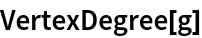Out=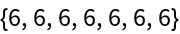The graph is semi-Eulerian and Eulerian:

 In:=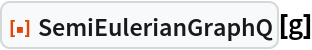Out=In:=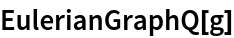Out=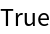A graph with more than two odd vertices:

 In:=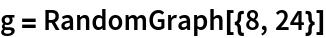Out=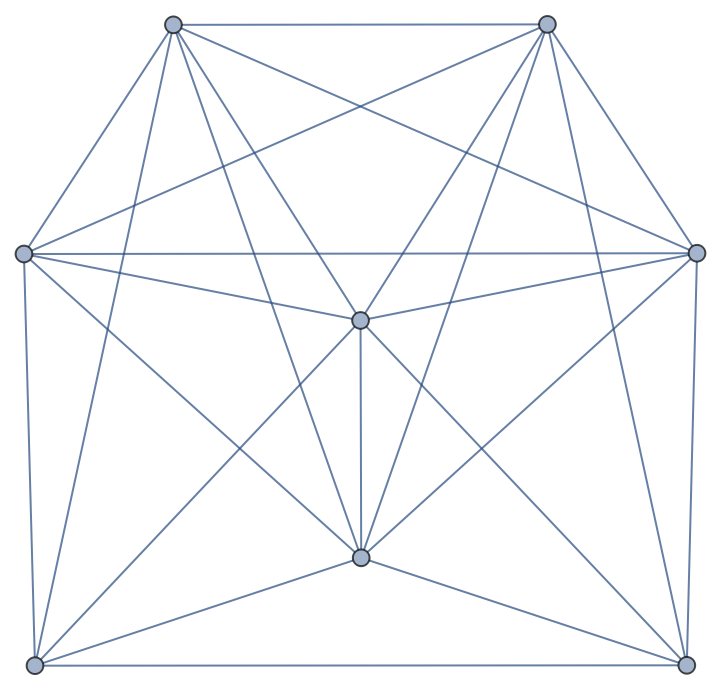In:=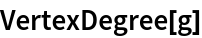Out=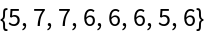The graph is neither semi-Eulerian nor Eulerian:

 In:=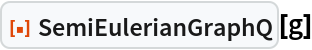Out=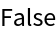In:=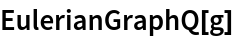Out=### Scope (2)

Test if a buckyball graph is semi-Eulerian:

 In:=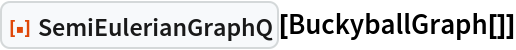Out=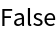Test if a torus graph is semi-Eulerian:

 In:=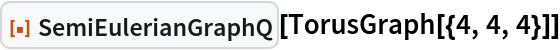Out=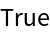### Neat Examples (1)

The multigraph representing the bridges of Königsberg is not semi-Eulerian:

 In:=Out=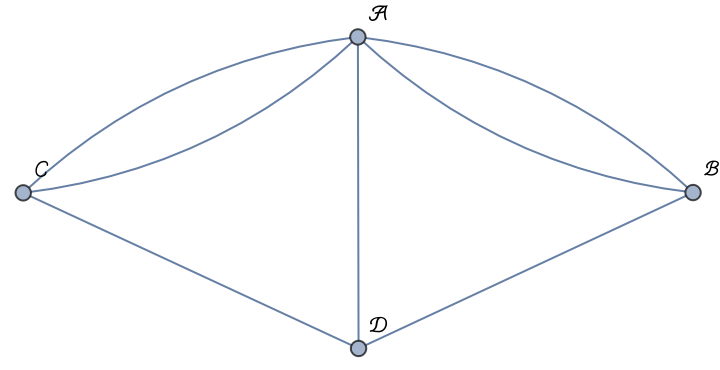In:=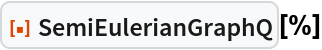Out=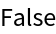Peter Burbery

## Version History

• 1.0.0 – 29 August 2022

## Author Notes

I might add a way to test if a directed graph and mixed graph is semi Eulerian in the future.